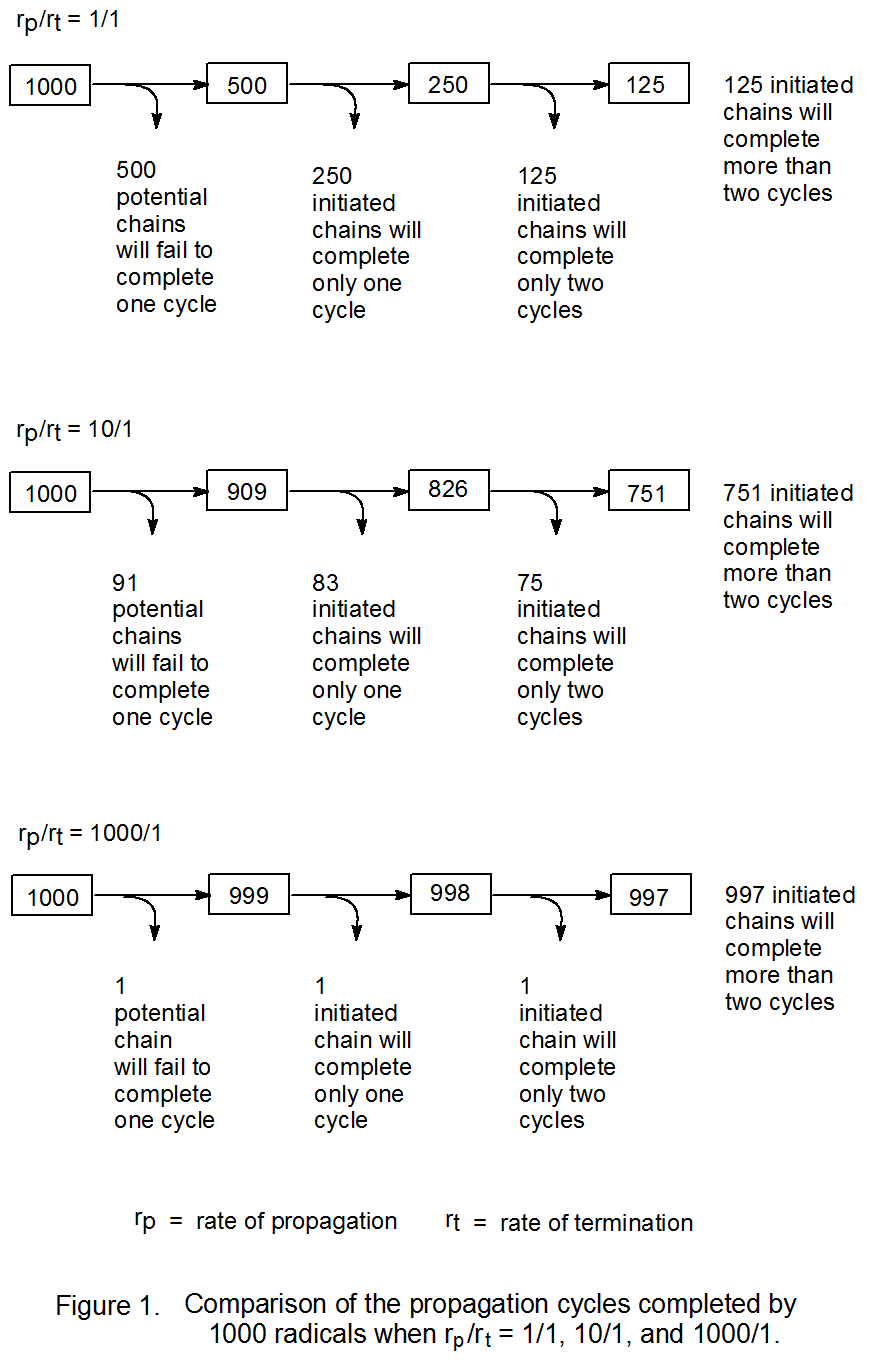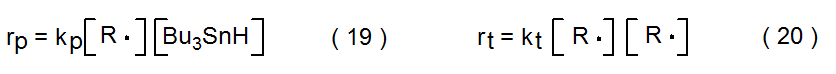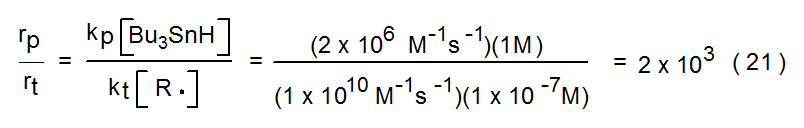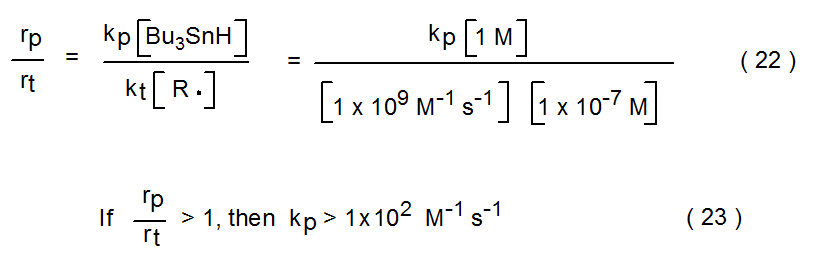# III. Reaction Efficiency

$$\newcommand{\vecs}{\overset { \rightharpoonup} {\mathbf{#1}} }$$ $$\newcommand{\vecd}{\overset{-\!-\!\rightharpoonup}{\vphantom{a}\smash {#1}}}$$$$\newcommand{\id}{\mathrm{id}}$$ $$\newcommand{\Span}{\mathrm{span}}$$ $$\newcommand{\kernel}{\mathrm{null}\,}$$ $$\newcommand{\range}{\mathrm{range}\,}$$ $$\newcommand{\RealPart}{\mathrm{Re}}$$ $$\newcommand{\ImaginaryPart}{\mathrm{Im}}$$ $$\newcommand{\Argument}{\mathrm{Arg}}$$ $$\newcommand{\norm}{\| #1 \|}$$ $$\newcommand{\inner}{\langle #1, #2 \rangle}$$ $$\newcommand{\Span}{\mathrm{span}}$$ $$\newcommand{\id}{\mathrm{id}}$$ $$\newcommand{\Span}{\mathrm{span}}$$ $$\newcommand{\kernel}{\mathrm{null}\,}$$ $$\newcommand{\range}{\mathrm{range}\,}$$ $$\newcommand{\RealPart}{\mathrm{Re}}$$ $$\newcommand{\ImaginaryPart}{\mathrm{Im}}$$ $$\newcommand{\Argument}{\mathrm{Arg}}$$ $$\newcommand{\norm}{\| #1 \|}$$ $$\newcommand{\inner}{\langle #1, #2 \rangle}$$ $$\newcommand{\Span}{\mathrm{span}}$$$$\newcommand{\AA}{\unicode[.8,0]{x212B}}$$

Reaction efficiency is a relative concept. Ref­er­ence to efficiency can take the form of a state­ment that one reaction is more efficient than another or that a reaction is an efficient pro­cess. Establishing a dividing line between efficient and inefficient reactions involves an arbi­trary decision; nevertheless, it is sometimes helpful in discussing chain reac­tions to use the term efficiency and to associate a numerical value with it. Choosing chain length to measure efficiency can provide this number; thus, one way to define an efficient reaction is as one that has a chain length greater than 100.65

The efficiency of a chain reac­tion is determined by its relative rates of propagation (rp) and ter­­min­ation (rt). A reaction becomes more effici­ent as the ratio rp/rt increases; thus, the chain length in a reaction differs sig­ni­fi­cantly when rp /rt is 10/1 as opposed to when it is equal to 1/1 (Figure 1). When rp /rt is 1/1, only 12.5% of the initiating radicals begin a chain des­tined to have a length greater than 2, but if the ratio of rpto rt is raised to 10/1, more than 75% of the initiating radicals produce chains with lengths greater than 2 (Figure 1). Even when rp/rt is 10/1, the reaction would not be des­cribed as an efficient one because a chain length of 100 in such a reaction would be a rare event. If, on the other hand, rp/rt is equal to 1000/1, nearly every initiated chain will complete two cycles (Figure 1) and most chains will have a length greater than 100; thus, the reaction is an efficient one. One desirable char­acteristic of a reaction with a long chain length is that con­verting all the starting material into product requires only a small amount of initiator.It is informative to consider some actual numbers for rates of pro­pa­ga­tion (rp) and termination (rt) reactions to better appreciate how these rates determine chain length. One way to do this is to analyze a typical reaction such as the dehalogenation process shown in Scheme 9. Since the rate deter­mining propagation step in this type of reaction is hydrogen-atom abstraction by R· from Bu3SnH,66,67 the rate of propagation (kp) is given by eq 19. A typical value for the rate constant (kp) for this reaction is 2 x 106 M-1 s-1.56 Since combination of two R· radicals is assumed to be the only significant ter­min­ation process, the rate of termination is given by eq 20. The rate constant for termination (kt) will depend upon how rapidly these radicals come together in solution, that is, upon their rate of diffusion. The rate constant for diffusion is 1 x 1010 M-1 s-1 in benzene,68 a common sol­vent for such reactions. A typical radical con­cen­tration in a chain reaction is less than 1 x 10-7 M.57,62 A normal concen­tration for a hydrogen-atom donor is one molar. Based upon these numbers and the assump­tions made about this reac­tion, dehalo­gen­ation with tri-n-butyltin hydride as the hydrogen-atom donor would be quite efficient because rp /rt would be approximately 2000 (eq 21).Although discussion of chain reactions naturally focuses on how to increase efficiency, it is informative to examine the other end of the effic­iency spectrum to determine the problems associated with inefficient reac­tions. As reactions become less efficient, more chains must be started in order for reac­tion to reach completion; consequently, more initiator must be added to the reaction mix­ture to compensate for the decrease in the average chain length. Increasing the initiator concentration increases the possibility of side reactions involving the initiator and radicals present in solution. As efficiency drops, chain termin­ation products become more abundant. When this hap­pens, product purifi­cation sometimes is more difficult. There are clear disadvantages to inefficient reac­tions.

A guideline for deciding when a chain reaction becomes too inefficient to be synthetically useful can be formulated in terms of rates of propagation and termination reactions.57 A cri­terion for usefulness is that the rate of propagation for a reaction (eq 19) should be greater than its rate of term­in­ation (eq 20).57 (This means that the first pos­si­bility pictured in Figure 1 would be just on the “wrong side” of the line for syn­thetic usefulness.) This dividing line is a reasonable one because any reaction in which an undesired product is formed at a faster rate than the desired product (rt > rp) is unlikely to be effective in synthesis.

Using the guideline that rp /rt should be greater than unity in a syn­the­tically useful reaction sets a lower limit on the rate constant for propagation (kp).57 This limit is based on the following assumptions: (a) chain term­in­ation is a diffusion controlled process for which the rate constant (kt) is between 1 x 109 and 1 x 1010 M-1 s-1;68 (b) the radical concentration in a typi­cal reaction is approximately 1 x 10-7 M;57,62 and (c) the concentration of the hydrogen-atom donor Bu3SnH is 1 M. Based on these assumptions, the rate con­stant for propagation (kp) for a synthetically useful reaction should be greater than 1 x 102 M-1 s-1 (eq 22 and eq 23). This limiting value for kp would hold for any reaction that satisfies the assumptions (a)-(c).This page titled III. Reaction Efficiency is shared under a All Rights Reserved (used with permission) license and was authored, remixed, and/or curated by .